# Samacheer Kalvi 6th Science Solutions Term 1 Chapter 2 Force and Motion

## Tamilnadu Samacheer Kalvi 6th Science Solutions Term 1 Chapter 2 Force and Motion Questions and Answers

### Samacheer Kalvi 6th Science Force and Motion Textual Evaluation

Force And Motion 6th Standard Question 1.
Unit of speed is
(a) m
(b) s
(c) kg
(d) m/s
(d) m/s

Force And Motion Class 6 Book Back Questions And Answers Question 2.
Oscillatory motion among the following is
a. Rotation of the earth about its axis
b. Revolution of the moon about the earth
c. To and fro movement of a vibrating string
d. All of these.
c. To and fro movement of a vibrating string

Unit 2 Force And Motion Question 3.
The correct relation among the following is
(a) Speed = distance × time
(b) speed = distance / time
(c) Speed = time / distance
(d) speed = 1/ (distance × time)
(b) speed = distance / time

Force And Motion Class 6 Question 4.
Gita rides with her father’s bike to her uncle’s house which is 40 km away from her home. She takes 40 minutes to reach there.
Statement 1 : She travels with a speed of 1 km/minute
Statement 2: She travels with a speed of 1 km/hour
a. Statement 1 alone is correct.
b. Statement 2 alone is correct
c. Both statement 1 and 2 are correct.
d. Neither statement 1 nor statement 2 is correct.
a. Statement 1 alone is correct.

II. Find whether the following statements are true or false. – if false give the correct answer.

6th Standard Science 2nd Lesson Question 1.
To and fro motion is called oscillatory motion.
True.

Samacheer Kalvi Guru 6th Science Book Back Answers Question 2.
Vibratory motion and rotatory motion are periodic motions.
False. Vibratory motion and oscillatory motion are periodic motions.

Samacheer Kalvi.Guru 6th Science Question 3.
Vehicles moving with varying speeds are said to be in uniform motion.
False. Vehicles moving with varying speeds are said to be in ton-uniform motion.
(OR)
Vehicles moving with uniform speeds are said to be in uniform motion.

Samacheer 6th Science Guide Question 4.
Robots will replace human in future.
False. Robots wili not replace human in future.

III. Fill in the blanks.

1. A bike moving on a straight road is an example of _______ motion.
2. Gravitational force is a _______ force.
3. Motion of a potter’s wheel is an example of _______ motion.
4. When an object covers equal distances in equal interval of time, it is said to be in _______ motion.

1. Linear
2. Non Contact Force
3. rotatory
4. uniform

IV. Match the following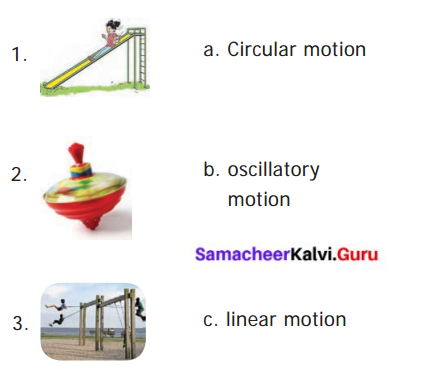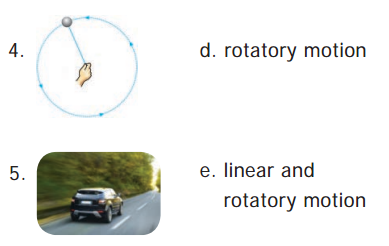1. c
2. d
3. b
4. a
5. e

V. Analogy

Samacheer Kalvi Guru 6th Standard Science Question 1.
kicking a ball: contact force :: falling of leaf: ________?
Non contact force.

Samacheer Kalvi Guru 6th Science Solutions Question 2.
Distance : metre :: speed : _______ ?
metre/second.

Samacheer Kalvi Guru 6 Science Question 3.
circulatory motion :: a spinning top :: oscillatory motion : _______ ?
Swinging of a pendulum.

VI. Given below Is the distance-travelled by an elephant across a forest with uniform speed. Complete the data of the table given below with the idea of uniform speed.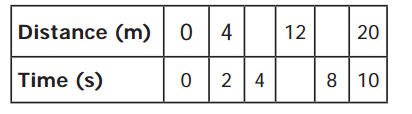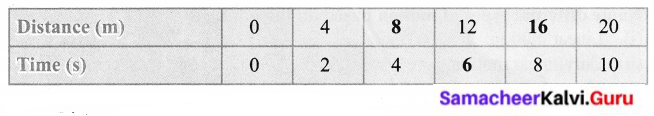(i) Distance/Time = $$\frac { 4 }{ 2 }$$ × 4 = $$\frac { 16 }{ 22 }$$ = 8
(ii) Distance / Time = $$\frac { 4 }{ 2 }$$ × 8 = $$\frac { 32 }{ 2 }$$ = 16
(iii) Time/Distance = $$\frac{1 \emptyset}{2 \emptyset}$$ × 12 = $$\frac { 12 }{ 2 }$$ = 6

VII. Complete the web chart.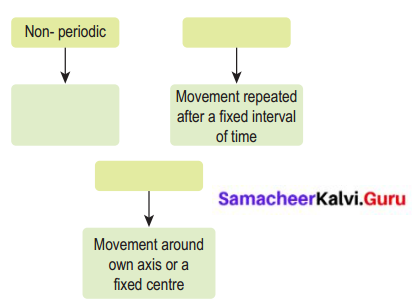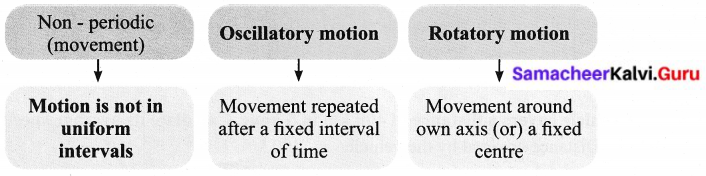VIII. Give one word for the following statements.

6 Samacheer Science Guide Question 1.
The force which acts on an object without physical contact with it.
non-contact forces.

Samacheer Kalvi Guru 6th Science Guide Question 2.
A change in the position of an object with time.
Motion.

Samacheer Guru 6th Science Question 3.
The motion which repeats itself after a fixed interval of time.
Oscillatory motion.

Samacheer Kalvi 6th Science Term 1 Question 4.
The motion of an object travels equal distances in equal intervals of time.
Uniform motion.

6th Science Solution Guide Question 5.
A machine capable of carrying out a complex series of actions automatically.
Artificial intelligence.

IX. Answer the following in a sentence or two.

Samacheer Kalvi 6th Science Solution Question 1.
Define force.
Forces are push or pull by an animate or inanimate agency.

Motion Book Back Answers Question 2.
Name different types of motion based on the path.

1. Linear motion;
2. Curvilinear;
3. Circular motion;
4. Rotatory motion;
5. Oscillatory motion
6. Irregular motion.

Class 6 Samacheer Science Solutions Question 3.
If you are sitting in a moving car, will you be at rest or motion with respect your friend sitting next to you?
I am in rest with respect to my friend, sitting inside the car.

6th Science Samacheer Guide Question 4.
Rotation of the earth is a periodic motion. Justify
Rotation of the earth is a periodic motion because it takes equal interval of time for all rotations.

Samacheer Kalvi 6 Science Question 5.
Differentiate between rotational and curvilinear motion.
Rotational motion:

1. A body moves along a circular path.
2. Without changing its position, about its own (fixed) axis.
3. Eg. Rotation of a spinning top.

Curvilinear motion:

1. A body moves along a curved path.
2. Changes its position with motion.
3. Eg. Throwing paper airplanes or paper darts.

X. Calculate

Samacheer Kalvi 6 Science Guide Question 1.
A vehicle covers a distance of 400 km in 5 hour. Calculate its average speed.
Distance covered by the vehicle = 400 km
Time taken = 5 hour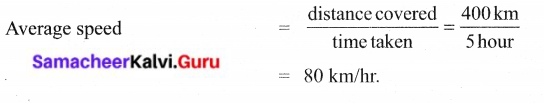6th Science Samacheer KalviQuestion 1.
What is motion? Classify different types of motion with examples.
Motion :
Change of position of an object with respect to time is known as motion.

1. Based on Path :

1. Linear motion. – Ex.: Parade of the soldiers.
2. Curvilinear motion. – Ex.: Paper flight moving.
3. Circular motion. – Ex.: Swirling stone tied to the rope.
4. Rotatory motion. – Ex.: Rotating top.
5. Oscillatory motion. – Ex.: Clock pendulum.
6. Zigzag (irregular) motion. – Ex.: Motion of a bee.

2. Based on Duration :

1. Periodic motion. – Ex.: Motion of a bob of simple Pendulum.
2. Non periodic motion. – Ex.: Swaying of the branches of a tree.

3. Based on Speed:

1. Uniform motion. – Ex.: Elour hand of a clock.
2. Non – uniform motion. – Ex.: Motion of a train, as it leaves a station.

XII. Fill with examples.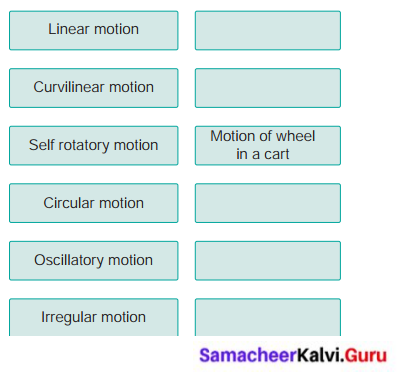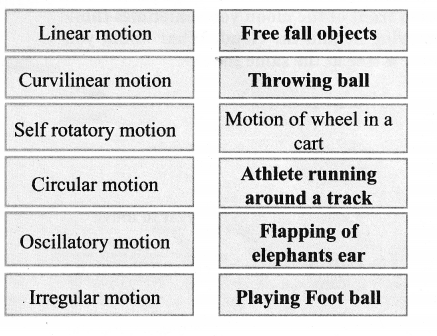### Samacheer Kalvi 6th Science Force and Motion Intext Activities

Activity – 1

Can you identify whether it is push or pull that results in motion in the following cases?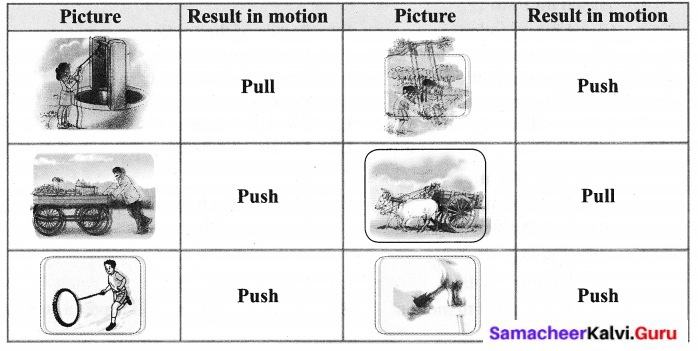Event 2:
The girl on the swing is moving with respect to the seat of the swing.
She is at rest with respect to the garden.

Event 3:
Nisha is going to her grandmother’s house by bicycle The girl on the bicycle is moving with respect to the road.
She is at rest with respect to the bicycle.

Activity – 2

Moon or Cloud?
Observe the moon on a windy night with a fair bit of cloud cover in the sky. As a cloud passes in front of the moon you sometimes think it is the moon which is moving behind the cloud. What would you think if you were to observe a tree at the same time?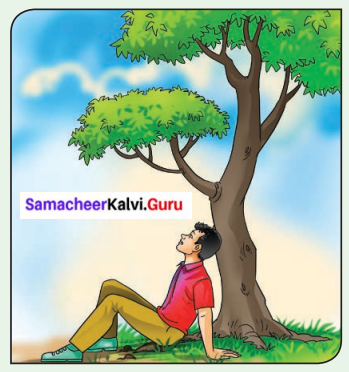Activity to be done by the students themselves

Activity – 3

Fill in the empty spaces.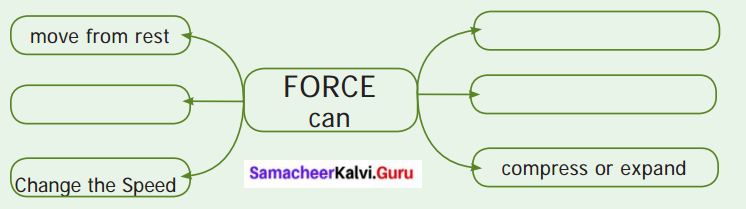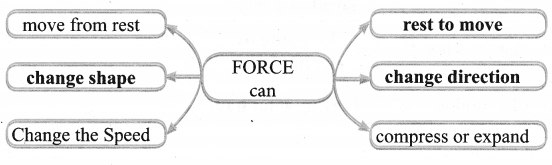Can you give example for contact and non-contact forces?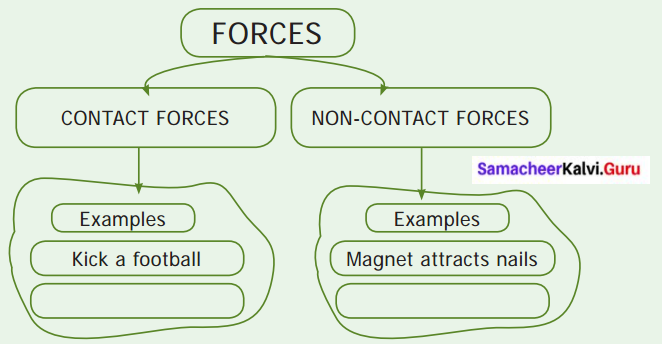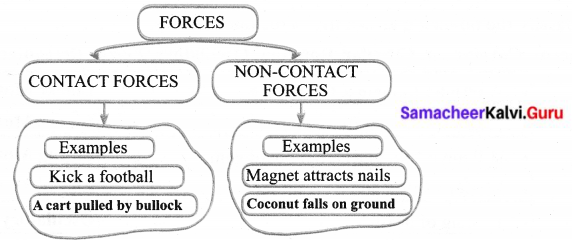Activity – 4
Play with pencil

1. Shanthi took a pencil and sharpened it with a sharpener.
2. Then she drew a circle using the pencil and a compass.
3. Later she took her ruler (scale) and drew a straight line in another paper.
4. Then she kept the pencil between her finger and moved it back and forth.Now, look at the motion of the pencil in all these four cases. How was it?

1. In the first case, the pencil rotated in its axis.
2. In the second case, it went in a circle.
3. In the third case, the pencil travelled in a straight line.
4. Fourth case, the pencil tip moved back and forth, that is it oscillated like a swing.

Activity – 5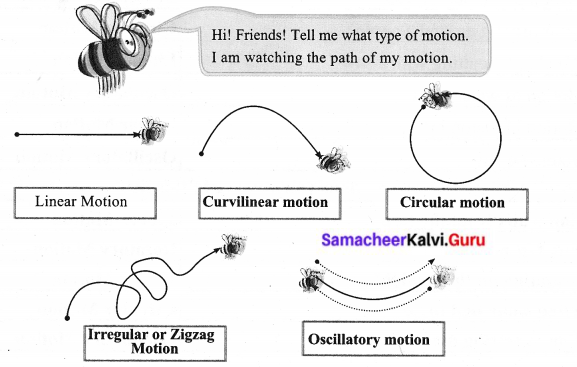Activity – 6
Classify the following according to the path it takes.

Linear, Curvilinear, Circular, Rotatory, Oscillatory, Zigzag (irregular)

• A sprinter running a 100 m race – Linear Motion
• A coconut falling from a tree – Linear Motion
• striking a coin in a carom board game – Zigzag Motion
• Motion of flies and mosquitoes – Zigzag Motion
• Beating of heart – Oscillatory Motion
• Children playing in a swing – Oscillatory Motion
• The tip of hands of a clock – Rotatory Motion
• Flapping of elephant’s ears – Oscillatory Motion
• A stone thrown into the air at an angle – Curvilinear Motion
• Movement of people in a bazaar – Zigzag Motion
• Athlete running around a track – Circular Motion
• Revolution of the moon around the earth – Circular Motion
• The movement of a ball kicked in a football match – Curvilinear Motion
• Motion of a spinning top – Rotatory Motion
• Revolution of the earth around the sun – Circular Motion
• Swinging of a pendulum – Oscillatory Motion
• Children skidding on a sliding board – Linear Motion
• Skidding down a playground slide – Zigzag Motion
• Wagging tail of a dog – Oscillatory Motion
• Flapping of a flag in wind – Oscillatory Motion
• A car driving around a curve – Circular Motion
• Woodcutter cutting with a saw – Linear Motion
• Motion of water wave – Oscillatory Motion
• Motion of piston inside a syringe – Linear Motion
• Bouncing ball – Oscillatory Motion

add five motions you observe to this list :

• Falling fruits from the tree – Linear Motion
• Ceiling fan running – Rotatory Motion
• Car running on the straight road – Linear Motion
• Swirling stone tied to the rope – Circular Motion
• Motion of sewing machine needle – Oscillatory Motion

Activity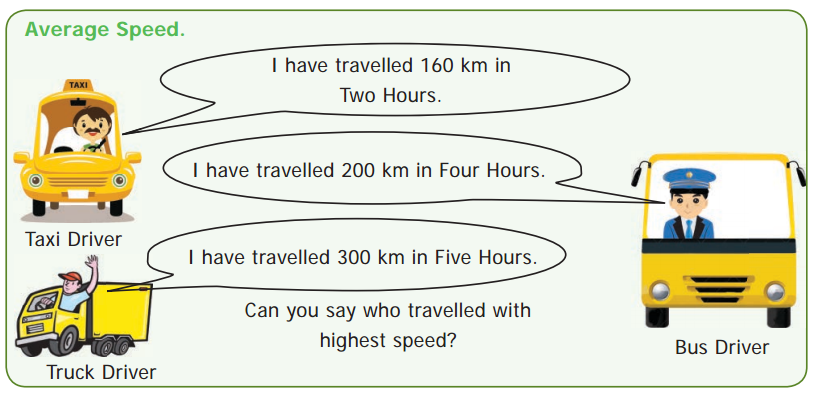The Taxi driver travelled with highest speed.

How do we say? Let us calculate how long they travelled in One Hour?
Distance travelled by the Car in One Hour = 80 km (160 ÷ 2)
Distance travelled by the Bus in One Hour = 50 km (200 ÷ 4)
Distance travelled by the Truck in One Hour = 60 km (300 ÷ 5)

Have you found out? say now.
Fastest Taxi, Slowest Bus.

Our speed…

Let us play a small game. Go to the playground with your friends. Mark 100 metre distance for a race. Conduct a friendly running race and calculate the time they taken to complete the distance by stopwatch. Now fill up the following table.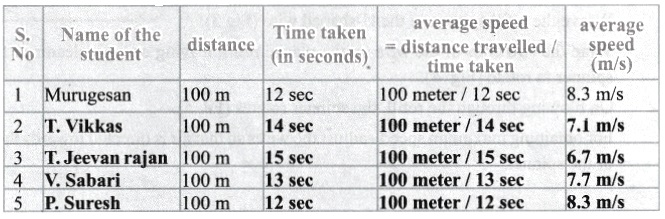Compute the following Numerical Problems.

1. If you travel 10 kilometres in 2 hours, your speed is 5 km per hour. (10 km/2 hours)
2. If you travel 15 kilometres in 1/2 hour, you would travel 30 km in one hour, and your speed is 30 km per hour. (15 km × 2/1 hours)
3. If you run fast at 20 kilometres per hour for 2 hours, you will cover 40 km. (20 km × 2 hours)

Activity

Multiple Motion to a Sewing MachineMotion of the needle
Periodic (or) Oscillatory motion

Motion of the wheel
Rotatory motion

Motion of footrest
Oscillatory motion

Activity – 7

Simple Spinner

Let us enjoy by making a simple spinner. Make it by the following instruction.

Cut a 2cm long piece from an old ball-pen refill and make a hole in its center with a divider point (Fig 1).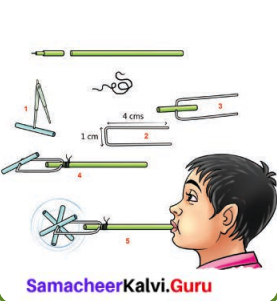Take a thin wire of length 9cm and fold it into a U-shape (Fig 2).

Weave the refill spinner in the U-shaped wire (Fig 3).

Wrap the two ends of the wire on the plastic refill, leaving enough clearance for the spinner to rotate (Fig 4).

On blowing through the refill, the spinner rotates (Fig 5).

For obtaining maximum speed – adjust the wires so that air is directed towards the ends of the spinner.

Have you enjoyed with simple spinner. Do you observe the motions in the toy. Can you answer the following question?

1. Motion of the air in tube is _____________ motion.
2. Motion of the refill stick _____________ motion.
3. The toy converts _____________ motion into _____________ motion.

1. Linear
2. Rotatory
3. Linear, Rotatory

### Samacheer Kalvi 6th Science Force and Motion Additional Questions

Question 1.
_______ is an ancient Indian astronomer.
(a) C.V. Raman
(b) Aryabhata
(c) Usain Bolt
(d) Edison
(b) Aryabhata

Question 2.
What is the path of the housefly buzzing around the room?
(a) Periodic motion
(b) Non- Periodic motion
(c) circular motion
(d) None of the above
(b) Non- Periodic motion

Question 3.
Identify the Periodic motion among the following :
(a) a horse running in a race
(b) revolution of the moon around the earth
(c) a coconut falling from a tree
(d) paper flight moving
(b) revolution of the moon around the earth

Question 4.
The cheetah the fastest animal among terrestrial animals run with the average speed of
(a) 100 km /hr
(b) 200 km/hr
(c) 112 km/hr
(d) 10 km /hr.
(c) 112 km/hr

Question 5.
_______ are robots scaled down to microscopic size in order to put them into very small spaces to perform a function.
(a) Car robots
(b) Home robots
(c) Game robots
(d) Nanobots
(d) Nanobots

Question 6.
A _______ is the fastest land animal.
(a) Horse
(b) Lion
(c) Cheetah
(d) Tiger
(c) Cheetah

Question 7.
A cheetah can run with an average speed of _______
(a) 112 km/h
(b) 121 km/h
(c) 211 km/h
(d) 122 km/h
(a) 112 km/h

II. Find whether the following sentences are true or false. If false Correct the statement.

Question 1.
Motion occurs when the object is pulled or pushed by an agency.
True.

Question 2.
Force executed by touching the body is called non – contact force.
False. Force executed by touching the body is called contact force.

Question 3.
Gravity pushes the ripen coconut from the tree to the ground.
False. Gravity pulls the ripen coconut from the tree to the ground.

Question 4.
Throwing paper aeroplane is the best example of linear motion.
False. Throwing paper aeroplane is the best example ofcurvilinear motion.

Question 5.
The movement of a body about its own axis like a rotating top is liner motion.
False. The movement of a body about its own axis like a rotating top is Rotatory

Question 6.
Motion repeated in equal intervals of time is called as periodic motion.
True.

III. Fill in the blanks.

1. _________ are push or pull by an animate or inanimate agency.
2. Application of force in an object results in motion from a state of _________
3. Fast oscillations are referred to as _________
4. Motion repeated in equal intervals of time is called _________
5. _________ are automatic machines.
6. The term Robots comes from a czech word _________
7. _________ is die study of robots in science.

1. Forces
2. rest
3. vibrations
4. periodic motion
5. Robots
6. robota
7. Robotics

IV. Complete the web chart.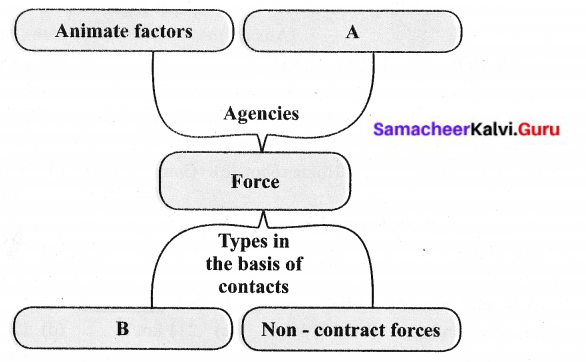• A – In animate factors,
• B – Contact forces.

V. Answer the following in one or two sentences.

Question 1.
What is meant by the State of Rest?
When there is no change of position of an object with respect to time and if it remains stationary it is called rest.

Question 2.
What is a non-contact force?
The force applied without touching the object is known as non-contact force.

Question 3.
What is the contact force?
When the force is in contact with the object then it is known as contact force.

Question 4.
Give the properties of force.

1. Forces can change the state of a body from rest to motion or motion to rest.
2. Forces can change the shape of the body.

Question 5.
A bus moves with a speed of 40 km and crosses 200 km and then how many hours has that bus taken to travel?
Time (t) = $$\frac{distence}{AverageSpeed}$$
Time (t) = $$\frac{200 km}{400 km/h}$$ = 5 hours

Question 6.
Define Average speed.
The distance travelled by an object in unit time is called average speed.Question 7.
List out the types of motion on the basis of speed.
There are two types:

1. Uniform motion,
2. Non-uniform motion.

Question 8.
Define uniform motion.
If an object covers uniform distances in uniform intervals, then the motion of the object is called uniform motion.

Question 9.
Why robots are used in many places?
Robots can perform mechanical and repetitive jobs faster, more accurately than human beings. It can also handle dangerous materials and explore distant planets.

Question 10.
What are the important parts of a robot?
Electronic sensors are a robot’s eyes and ears. These are the important parts.

Question 11.
What is nanobots?
Nanobots are robots scaled down to microscopic size in order to put them into very small places to perform a function.

Question 12.
What are the uses of future robots?

1. It could be placed in the bloodstream to perform surgical procedures.
2. It could target cancer cells and destroy them without touching healthy cells nearby.

Question 1.
Classify the following motions according to the path it takes.
(a) A coconut falling from a tree
(b) Heart beat
(c) A stone thrown into the air at an angle
(d) Movement of people in a bazzar
(e) Motion of a spinning top
(a) A coconut falling from a tree – Linear Motion
(b) Heart beat – Oscillatory Motion
(c) A stone thrown into the air at an angle – Curvilinear Motion
(d) Movement of people in a bazar – Zigzag Motion
(e) Motion of a spinning top – Rotatory Motion

Question 2.
Classify the following motions based on duration and speed.
(a) Coconut falls to the ground
(b) A cart pulled by a bullock
(c) Train journey
(d) A bouncing ball
(e) Revolution of Moon around the earth